When we apply a current to a trace, the trace heats up. It is possible to apply enough current to a trace that the temperature increases to the melting (fusing) point of the copper. We call this amount of current the fusing current. Recall from my previous article  that heating is a point concept. That means when a trace melts, it will do so at the weakest (probably thinnest) point along the trace.

If we want to calculate the fusing current for a trace, there are few ways to do it (short of a computer simulation.) Sir William Henry Preece (consulting engineer and later engineer-in-chief for the British General Post Office) developed an equation during the 1880s that has now become known as Preece’s Equation:

[Eq. 1]   I = 12277 * A3/4

Where:
I = fusing current in Amps
A = Cross-sectional area in inches

Later, I. M. Onderdonk developed a more complicated equation that introduces a variable for time: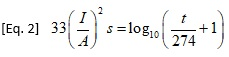Where:
I = the current in Amps
A = the cross-sectional area in circular mils
s = the time in seconds the current is applied
t = the rise in temperature from the ambient or initial state (assumed to be 40 degrees C)

Preece conducted his experiments in a laboratory. He assumed he had reached the fusing temperature when the wire under study began glowing. Onderdonk is believed to have derived his (her) equation, probably in the 1920s. The time frame for validity of Onderdonk’s Equation is usually given as under 10 seconds, since this equation assumes there is no cooling effect. For a discussion of what is known about these two people and for references to possible source documents, see .

Consider two possible scenarios:

A. A large sudden current (significantly larger than the fusing current) is applied to a trace. We would expect the current to significantly overload the trace, increasing the temperature, and melting a point on the trace quickly.

B. A current just larger than the fusing current is applied to the trace. The temperature will rise more slowly, ultimately melting the trace at its weakest point.

Figures 1 and 2 illustrate these two scenarios. Which figure do you think goes with which scenario ?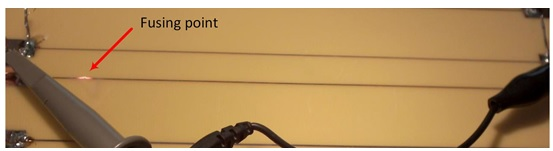Figure 1. Trace melting as a result of an applied current.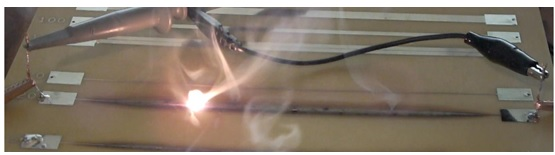Figure 2. Another trace melting as a result of an applied current.

If we apply a sudden, very large current across a trace, the trace heats very quickly. The trace continues to heat until it melts at some point, which (of course) stops the current flow immediately. In one experiment, we applied a sudden current of 6 Amps across a 15 mil wide, 0.5 Oz. trace. The graph of temperature vs time is shown in Figure 3. The trace melted (fused) in 2.75 seconds.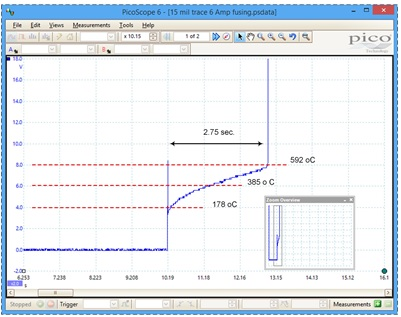Figure 3.  15 mil wide, 0.5 Oz trace, carrying 6 Amps.

The horizontal axis in the figure is time. The vertical axis is volts across the trace. Converting volts to temperature is problematic. What we know is the initial resistance across the trace, Rt0 , the initial temperature of the trace, the constant current through the trace, and, therefore, the initial voltage across the trace. We also know (we hope) the thermal coefficient of resistivity of the copper, α. Given these parameters, we can infer the temperature of the trace at any point in time by (first) measuring the voltage across the trace, estimating the trace resistance at temperature from Ohm’s Law, and finally calculating: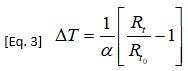Where:
ΔT   = change in temperature from ambient (ref) (oC)
α     = thermal coefficient of resistivity of copper (1/oC)
Rt    = resistance of the trace at the temperature under test (Ohms)
Rto = resistance of the trace at the reference temperature (Ohms)

The problems with this approach are at least three-fold:

1. Things are occurring so rapidly it is difficult to get uniformly precise measurements,
2. The estimated trace temperature is an average across the entire trace. As we know from the reference in , traces do not heat uniformly.
3. Since there are thermal gradients along the trace, then α itself will vary along the trace

Therefore, the temperature estimates shown on the graph are approximate (best guess) estimates.

The point of fusing for this type of situation is underwhelming. It is shown in Figure 1. The fusing time occurs within one video frame, or 1/30 second. There is no detectable smoke or damage to the board (other than the melted trace at a point).

If we apply a current to a trace that is approximately equal to the fusing current, the situation is quite different. On one of our test boards, we had a 20 mil wide, 1.5 Oz. thick trace. We applied 8.3 Amps of current to it. The temperature of the trace rose quickly to about 213 oC and then stabilized for over two hours. The next day we applied 8.5 Amps to the same trace. Figure 4 illustrates the pattern of temperature through time that resulted.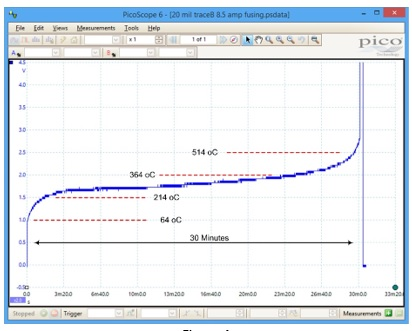Figure 4.  20 mil wide, 1.5 Oz. trace carrying 8.5 Amps.

As before, the temperature rose quickly to about 214 oC. But then it continued to increase slowly. After about 30 minutes the temperature had increased to about 514 oC, at which point the temperature began rising quickly to the point where the trace melted. The entire process took about 30 minutes . Figure 2 is from a video of this illustration, at about the moment of fusing. There are several board material thermal parameters that are relevant here : Tg: Glass Transition Temperature; Td: Thermal Decomposition Temperature; and Delamination Temperature(s) and Times.

As the temperature of the board reaches each of these thresholds, the effectiveness of the cooling process diminishes, and the rate of heating of the board increases. The threshold points are not easy to identify in Figure 4, but it is not hard to imagine how the process is working.

This process can be impressive to watch. At about 15 minutes, the aroma of burning dielectric began to permeate the room. At about 20 minutes the board began to smoke. At about 25 minutes smoke was exiting out from under the trace at several points, and at some of those points the ejection of the smoke was under some considerable pressure. Finally, a point along the trace erupted in flame as the trace melted. At that point, the circuit opened and the current flow stopped. There was obviously considerable damage done to the board along the trace where the overheating occurred.

(Aside: The melting process itself make take several forms. The trace may vaporize relatively quickly, breaking the circuit. The trace may melt, but the melted copper remains in place, allowing the current to continue flowing for some indeterminate time. Or, the trace may melt, and the melted copper floats away (perhaps through gravity) breaking the circuit.)

An interesting question is whether we can predict this fusing time. In the significant overload case (Figures 1 and 3), the answer is a qualified yes. Onderdonk’s Equation assumes there is no cooling process. References suggest that the equation applies for cases under about 10 seconds. But there is evidence that Onderdonk was concerned with (power transmission) wires suspended in the air. Traces on a board will cool much more quickly and efficiently. Our studies suggest that Onderdonk’s Equation will apply on a circuit board for up to 1.0 to 1.5 seconds. There are approximations we can apply to extend this time to perhaps up to 4.0 seconds .

### Notes:

2. See “PCB Trace and Via Currents and Temperatures: The Complete Analysis, 2nd Edition,” 2017, Douglas G Brooks, PhD and Dr. Johannes Adam, available on Amazon.com. Chapter 9 provides an extensive discussion about what is known about Preece and Onderdonk, as well as a derivation of Onderdonk’s Equation.
3. These figures are reprints of Figures 11.2 and 11.4 from studies reported in Chapter 11 of our book, referenced in Note 2.
4. The time for this process is unpredictable. It depends on the unique characteristics of the trace under test.
5. See pp. 16-17 in the reference in Note 2.
6. See Chapter 10 in the reference in Note 2.